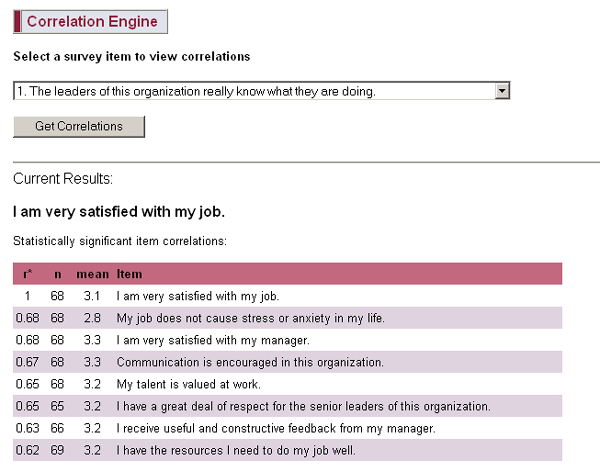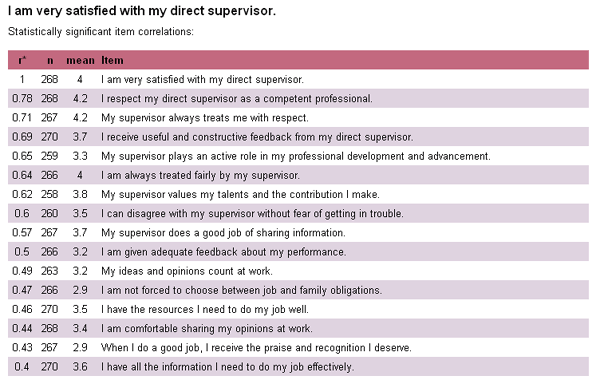Home > Articles > Correlation Analysis

# Correlation Analysis for Surveys

Correlation is a rather technical statistical concept - we're going to avoid most of the technical discussion here and just present some practical applications for using correlation to better understand survey results. This explanation is intended to help the layperson understand the basic concept of correlation without requiring a lot of technical knowledge.

We use correlation to help understand what drives employee satisfaction or employee engagement within organizations. The same technique can also be used for customer satisfaction or other types of surveys as well.

Correlation is a statistic that measures the linear relationship between two variables (for our purposes, survey items). The values for correlations are known as correlation coefficients and are commonly represented by the letter "r". The range of possible values for r is from -1.0 to +1.0. Numbers less than zero represent a negative relationship between variables and numbers greater than zero represent a positive relationship. You can think of the value of r almost like a percentage.

This screen shot (see below) of the output from our Correlation Engine shows how you might use correlation for an employee satisfaction survey. You can select any Likert rating scale item (e.g. 5-point rating) from your survey and view all the statistically significant correlations with that item. In this example, we are looking at the survey questions that are most correlated with overall employee satisfaction.

 From a statistical perspective, we have to make one disclaimer. Correlation cannot determine cause and effect. Strictly speaking, correlation can only indicate the strength of the statistical relationship between two survey questions. It cannot indicate which of those items is influencing the other item. (And in some cases, there could even be a third, unmeasured factor that is the real cause of the observed correlation between two survey items.) For example, take the item related to job stress and anxiety. There is no way to say for sure that employee satisfaction is a result of low stress, or the other way around - that low stress is a result of employee satisfaction. If you are feeling brave and you want to better understand correlation and causation, see Wikipedia's synopsis. Within the context of an employee satisfaction survey or an employee engagement survey, we take a morepragmatic approach. We assume that overall satisfaction or engagement is the effect, and that any survey questions that correlate with these concepts are the cause. This is a logical and safe assumption for overall satisfaction, especially if the survey covers a comprehensive list of areas related to employee satisfaction. However, when looking at correlations between other survey questions, it is important to keep the cause-effect uncertainty in mind. Logic will often tell you which is the cause and which is the effect, but not always.
Statistical Significance (p-level) and Number of Respondents ("n")

When you look at correlations, you will usually see something like the following in a footnote somewhere:
Correlations (r) significant at p < 0.05. This is a customary indication of the likelihood that the observed correlations are a result of chance. For our purposes, we have set this probability (p) threshold to be no more than 0.05 or 5%. There is less than a 5% likelihood that the correlations listed here are a result of chance.
Whenever you view correlations, it is important to look for this p-level. You don't need to understand more about it than is explained here. Just know that "p < 0.05" is the most common standard threshold for statistical significance.

"n" indicates the total number of respondents. This is important for statistical significance because when you have a large n, a smaller correlation can still be statistically significant. Conversely, with a small n, you need a much larger correlation for statistical significance. If you are looking at two sets of correlations that have very different numbers of respondents, you can NOT compare the correlation coefficients from each list to one another. You need to look at each list independently and draw conclusions only within each list.

In the example above, the correlations are pretty close to one another in value. Notice in the example below how there are bigger gaps between the correlations. When you see a couple of items at the top with much higher coefficients (r) than the others and then a big drop in r for the following items, focus your attention more on those top items. If you have several items that are close to one another, you should still start at the top of the list, but give more equal weight to the items that follow the top items. There is often a natural cut-off point somewhere in the list where you will see a big drop in r - use this as a logical point to limit your analysis.In this second example, there is a big gap after the first item, so we might conclude that the number one factor that determines whether people are satisfied with their supervisor is competence. We would also want to look at the second and third items since these are still strong correlations and provide useful additional information. In fact, we would probably want to consider all the items down to the next big drop in r where it goes from 0.57 to 0.50. At this point, we have about as many items as we can deal with - the remaining items are still of interest, but should not be focused on too closely.

The most common way that correlation is used in most surveys is to find out what matters most to people by correlating survey items with some measure of overall satisfaction. As you've seen in the examples above, this is a technique that you can safely use without worrying about all the technical stuff. We filter out all the noise and just show you those correlations that are statistically significant. You just start at the top of the list to see what matters most. (Remember to also look at the bottom of the list - high negative correlations, while less common, are just as important as high positive correlations. A negative correlation indicates an inverse relationship between items.)

More on using correlations to measure employee satisfaction

 Tweet